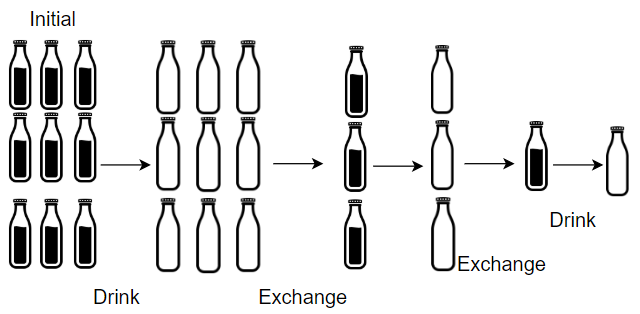1518. Water Bottles

# 1518. Water Bottles#

## 题目 #

Given numBottles full water bottles, you can exchange numExchange empty water bottles for one full water bottle.

The operation of drinking a full water bottle turns it into an empty bottle.

Return the maximum number of water bottles you can drink.

Example 1:``````Input: numBottles = 9, numExchange = 3
Output: 13
Explanation: You can exchange 3 empty bottles to get 1 full water bottle.
Number of water bottles you can drink: 9 + 3 + 1 = 13.
``````

Example 2:``````Input: numBottles = 15, numExchange = 4
Output: 19
Explanation: You can exchange 4 empty bottles to get 1 full water bottle.
Number of water bottles you can drink: 15 + 3 + 1 = 19.
``````

Example 3:

``````Input: numBottles = 5, numExchange = 5
Output: 6
``````

Example 4:

``````Input: numBottles = 2, numExchange = 3
Output: 2
``````

Constraints:

• 1 <= numBottles <= 100
• 2 <= numExchange <= 100

## 解题思路 #

• 模拟。首先我们一定可以喝到 numBottles 瓶酒，剩下 numBottles 个空瓶。接下来我们可以拿空瓶子换酒，每次拿出 numExchange 个瓶子换一瓶酒，然后再喝完这瓶酒，得到一个空瓶。这样模拟下去，直到所有的空瓶子小于numExchange结束。

## 代码 #

``````package leetcode

func numWaterBottles(numBottles int, numExchange int) int {
if numBottles < numExchange {
return numBottles
}
quotient := numBottles / numExchange
reminder := numBottles % numExchange
ans := numBottles + quotient
for quotient+reminder >= numExchange {
quotient, reminder = (quotient+reminder)/numExchange, (quotient+reminder)%numExchange
ans += quotient
}
return ans
}
``````Nov 25, 2022Edit this page